BIOL245 General Ecology Exam #2 KEY

Fall 1998

Your answers to these questions should be complete, yet succinct. Extraneous material will count against you. Show all work for the problems, and label the axes of your graphs. Each correct answer is worth five points unless otherwise noted.

1. Define and illustrate graphically population regulation.

Regulation is homeostasis, which in populations is the negative feedback between density (N ) and growth dN/dt. Thus, the most direct way to illustrate population regulation is by showing the theoretical relationship between these two variables: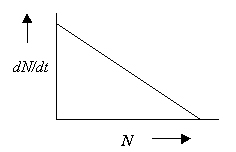2. Using the predictive form of the exponential growth equation, predict population size in ten years of 150 turtles growing at 0.01 (1%) annual per capita rate of increase. How long would it take the population to double from 150?
The predictive solution to the exponential growth equation dN/dt = rN is:

Nt = N0ert

more easily solved as: lnNt = lnN0 + rt

Now we substitute, where N0 = 150; r = 0.01; t = 10: lnN10 = ln150 + 0.01(10)

which gives the answer lnN10 = 5.11, so N10 = 166 (rounded up)

Now, to solve for doubling time from 150 to 300, solve the equation for t:

t = ( lnNt - lnN0)/r

t = 69+ years

3. How does the Malthusian parameter r reflect an organism's position in the fundamental niche? Show the position on a niche axis (your choice) of an organism growing according to its biotic potential.
The parameter r can be interpreted as mean fitness for a population. If the population is growing according to this biotic potential, most individuals must be near the optimum position in the fundamental niche of that species. For any given niche factor, there is a tolerance range, and the optimum place on that range is defined as that which gives the best fitness, reflected by the greatest frequency: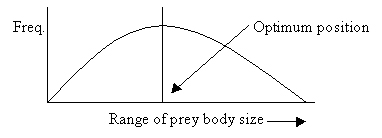4. How fast is a logistic population of 40 individuals growing if r = 0.02 and the carrying capacity of the environment is 250?
A logistic population has the sigmoid growth form generated by the equation:

dN/dt = rN(1 - N/K)

and the growth with the above numbers would be:

dN/dt = 0.02(40)(1 - 40/250)

= 0.67

5. What is the significance of the inflection point on the logistic growth curve? Show the inflection point on a graph where x = density and y = dN/dt.
The inflection point, where Nt = K/2, is the point at which the population replaces itself at the maximum rate, i.e., maximum dN/dt. This is the "maximum sustainable yield" for exploited populations such as fish stocks, or the population density of pests that is hardest to control.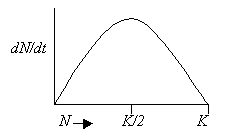6. What are the assumptions for logistic growth, and how realistic are they?
Logistic growth assumes that a population will be responsive to the carrying capacity of the environment ("K -recognition"), which is an evolved trait that can be demonstrated in nature for real populations. It also assumes that every individual added to the population has the same impact on growth as every other individual, which implies that all individuals take equal portions of the resource and that adding individuals at low density has the same impact on growth as adding individuals at high density. Since low density populations are unlikely to be resource limited, and since different ages, sexes, and reproductive conditions of individuals will cause variability in resource demand, these assumptions are unrealistic. Finally, r and K are assumed to be constant. Biotic potential may be constant over several generations, but carrying capacity of the environment can vary stochastically in the short term. In spite of these limitations, the logistic is a good ideal model with which to compare real K - responsive populations, because deviations from the ideal sigmoid growth curve can tell us which assumptions are violated.

7. What is the form of the global human population curve since the Middle Ages? Explain how this came to be. What is global carrying capacity for humans?
The growth curve of humans on a global scale has been exponential. Much of this can be explained by raising the birth rate and carrying capacity through agriculture and industry, and lowering death rate by improvements in medical care. Establishment of a numerical value for global carrying capacity is a thorny issue, but a reasonable standard of living would put the value between one and two billion people, or about a third of the current population size.

8. What is sexual selection, and how does the "handicap hypothesis" fit into this idea?
The ability of an individual to choose a mate based on heritable behavioral or morphological traits involved in mating behavior constitutes sexual selection. It is like natural selection, in that an individual's fitness depends upon how well it can attract a mate relative to others in the deme, with mate choice being the selective agent. The handicap hypothesis assumes that secondary traits that make one sex (usually male) more conspicuous to the other also make it more attractive to predators. Thus, the female, by choosing the conspicuous male, selects for a handicap in terms of escape from predation.

9. What features of an oak tree are r-selected? Which features are K-selected?
An oak tree is r-selected in that it has a large brood size (seed set) and Type III survivorship. It is K-selected in that it inhabits mature communities in which competition is important, has long generation time and large body size.

10. Explain "parental investment."
Parental investment is the proportion of an organism's energy budget expended on reproduction. It can involve expenditure on mating behavior (courtship, territoriality, etc.), care of offspring, and number of offspring. The trade-offs between semelparity and iteroparity can also be interpreted as different strategies of parental investment: whether to put all your eggs in "one basket" or to spread out reproduction over several breeding seasons. These trade-offs are illustrated by the mathematical relationship of brood size and age of first reproduction on the relative difference between semelparity and iteroparity in terms of fitness (r).

11. Explain and graphically illustrate on a single pair of axes the relationships among age of first reproduction, brood size, and r for an iteroparous organism.
The longer first reproduction is delayed the lower r is, and the less difference brood size makes in r: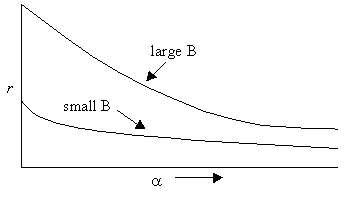12. Define competition, and differentiate between interference and exploitation.

Competition occurs when two or more organisms exploit the same, limiting, resource. This interaction negatively affects all competitors, but not necessarily to an equal degree (i.e., most competition is asymmetrical). Competition can be intraspecific or interspecific. Interference occurs when "access to a resource is limited by a the presence of a competitor" (from Smith's text), which means both competitors are present at the same time in the same place. This can result in "scramble" competition, in which none of the participants is able to acquire sufficient resource to survive. Exploitation, or "niche preemption," occurs when one competitor makes the resource (usually space) unavailable to its competitors, usually by being there before the others. Intraspecific competition is mainly by interference.
13. What is the Competitive Exclusion Principle? How does the notion of guilds relate to this idea?
If competing species have identical resource requisites, eventually the inferior competitor will go extinct. The greater the differences in exploitation (methods or subsets of a resource), the lower the intensity of competition. Guilds are groups of species that exploit the same class of resources, but generally are to some degree dissimilar in their preferred methods, area of foraging, or size class of resources. These differences allow coexistence, but whether they arise as the "ghost of competition past" or are merely preadaptive is usually undetermined.

14. How does disturbance from predators or other extrinsic agents affect competition, and what has this to do with the number of species that can coexist in a community? Show this graphically.
Disturbance reduces competition, because it lowers population densities of competing species relative to the amount of limiting resource (i.e., makes the resource less limiting). The intermediate disturbance hypothesis suggests there is an optimal level of disturbance that allows a maximum number of potentially competing species to coexist. Less than this level of intensity allows competition to reduce diversity; greater than this level constitutes "overgrazing" which can cause extinction.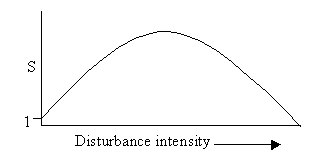15. One of the outcomes of the Lotka-Volterra competition model is "either species wins, depending." Depending upon what?
The outcome of competition can depend upon patterns in the environment that may favor one species in one location, and the other in another location; similarly, the environment may shift in time to favor first one and then the other (e.g., seasonality, diurnality, etc.). The outcome can also be affected by which species colonizes the resource first, and how much time elapses before the others get there. An inferior competitor can win an interaction if it is allowed to become well-established with a large enough population before its competitors arrive.

16. What is a competitive network?
Competitive networks, sometimes called non-transitive competition, are groups of species for which the outcomes of competition are not linear or hierarchical. One species may outcompete a second species, the loser may in turn win over a third species, but the third species can outcompete the first. This may result from a difference in competitive strategies and susceptibilities among species. It is uncertain how important this kind of interaction is in nature; most demonstrations have involved space as the limiting resource.

17. Explain how intraspecific and interspecific competition affect niche width of a species population.
Intraspecific competition should force less fit members of a population to seek sub-optimal resources, thus widening the repertoire for the population as a whole: niche width increases. The mating system of the grouse is an example, wherein less fit males are forced to display for mates on the periphery of the optimal spatial position in the lek. Essentially, intraspecific competition means there is not enough of the optimal resource to go around, so inferior individuals must seek lesser portions of that niche factor. Interspecific competition, by contrast, produces greatest selection against sub-optimal individuals in the zone of overlap among competing species; hence, niche width is decreased. The shape of the resource niche at any given time thus depends on a balance between these two opposing forces.

18. Write the general growth equation for one of two species in Lotka-Volterra competition. Show and describe where intraspecific and interspecific competition are accounted for.
The Lotka-Volterra equation is a simple modification of the logistic equation to account for the effect of a second species on the growth of the first. In the logistic, the term
(K-N)/K is a measure of intraspecific competition, because it reflects the negative effect of increasing density on growth as density gets closer and closer to carrying capacity. In order to measure the effect of a second species, we need to correct for probably inequities in the impact of different species on the resource, hence on the growth of the first population. This is done by subtracting the number of individuals of the second species, modified by a competition coefficient, a = the relative impact of each individual of species 2 on the growth of species 1:

dN1/dt = r1N1(1 - N1/K - a N2/K)

19. (10 points!) Draw the competition isoclines and predict the outcome of competition between species 1 (K = 250; a = 1.4) and species 2 (K = 1,500; b = 0.9).
We first need the relationship between carrying capacities and competition coefficients, in order to establish intercepts for both axes:

K1/a = 179; K2/b = 1666, so K1/a < K2 and K2/b > K1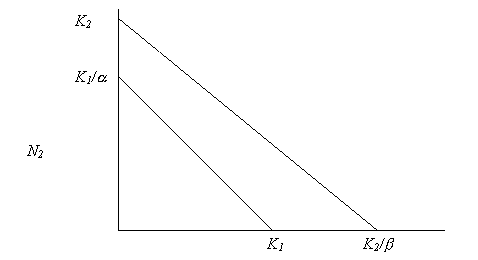Since the isocline defining dN2/dt is everywhere above the isocline for species 1, species 2 wins, no matter what the initial conditions.